×#### Thank you for registering.

One of our academic counsellors will contact you within 1 working day.

Click to Chat

1800-1023-196

+91-120-4616500

CART 0

• 0

MY CART (5)

Use Coupon: CART20 and get 20% off on all online Study Material

ITEM
DETAILS
MRP
DISCOUNT
FINAL PRICE
Total Price: Rs.

There are no items in this cart.
Continue Shopping• Complete JEE Main/Advanced Course and Test Series
• OFFERED PRICE: Rs. 15,900
• View Details

```Chapter 8: Division of Algebraic Expressions Exercise – 8.4

Question: 1

Divide 5x3 - 15x2 + 25x by 5x.

Solution:Question: 2

Divide 4z3 + 6z2 – z by – (1/2)z.

Solution:Question: 3

Divide 9x2y - 6xy + 12x2 by – (3/2)xy.

Solution: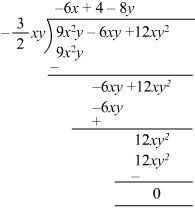Question: 4

Divide 3x3y2 + 2x2y + 15xy by 3xy

Solution: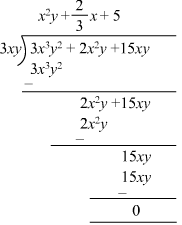Question: 5

Divide x2 + 7x + 12 by x + 4.

Solution: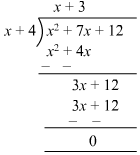Question: 6

Divide 4y2 + 3y + (1/2) by 2y + 1.

Solution: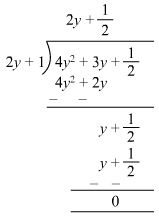Question: 7

Divide 3x3+ 4x2+ 5x + 18 by x + 2.

Solution:Question: 8

Divide 14x2– 53x + 45 by 7x – 9.

Solution:Question: 9

Divide – 21 + 71x – 31x2 - 24x3 by 3 - 8x.

Solution: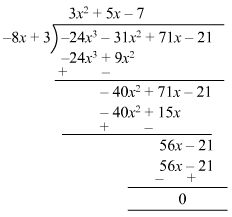Question: 10

Divide 3y4 – 3y3 – 4y2 – 4y by y2 – 2y.

Solution: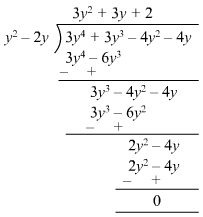Question: 11

Divide 2y5 + 10y4 + 6y3 + y2 + 5y + 3 by 2y3

Solution: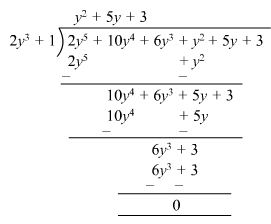```### Course Features

• 728 Video Lectures
• Revision Notes
• Previous Year Papers
• Mind Map
• Study Planner
• NCERT Solutions
• Discussion Forum
• Test paper with Video Solution